Introduction to Decimals 2

 Problem Set 1 Problem Set 2 Problem Set 3Select a problem set using the buttons above, then use your mouse or
tab key to select a question. Fill in the blank with the correct answer.

Use '/' to express the answers which are fractions.
Make sure to use commas where appropriate.

 Example: 7 should be written as 7/1,000 1,000

When you have answered all of the questions, ask Charlie how you did.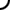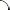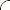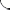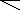Write each fraction as a decimal.

Write each decimal as a reduced fraction.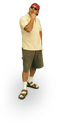Write each fraction as a decimal.

Write each decimal as a reduced fraction.Write each fraction as a decimal.

Write each decimal as a reduced fraction.©  2009  LarryPerezWeb Design:  Patrick Quigley